## Given problem

Suppose we have an array, we need to sort this array followed by increasing order.In this article, we will use binary insertion sort to deal with this problem.

## How Binary Insertion Sort works

Steps of Binary Insertion Sort are similar to Insertion Sort. But to insert a new element into a sorted subarray, we use binary search algorithm to find the suitable position for it, instead of iterating all elements backward from i to 0 position.

Belows are some steps that describe Binary Insertion sort works.

1. Original array

2. Insert an element at index `i` = 1 into a sorted subarray has `length = 1`, from the `position = 0`.

• Use binary search to find position –> `pos = 1`.

• shift all elements that are from `pos` to `i - 1` to the right side by one position.

Because an element at index = 1 always satisfies in the sorted array. So, we have our array: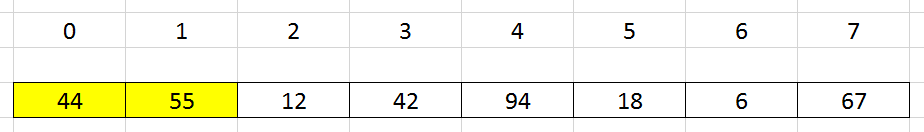3. Insert an element at index `i` = 2 into a sorted subarray has `length = 2`, from the `position = 0`.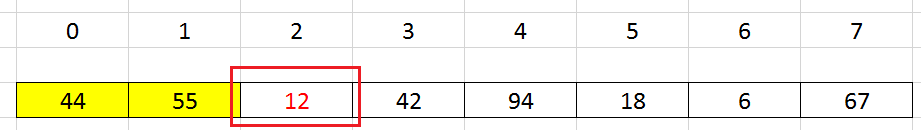• Use binary search to find position –> `pos = 0`.

• shift all elements that are from `pos` to `i - 1` to the right side by one position.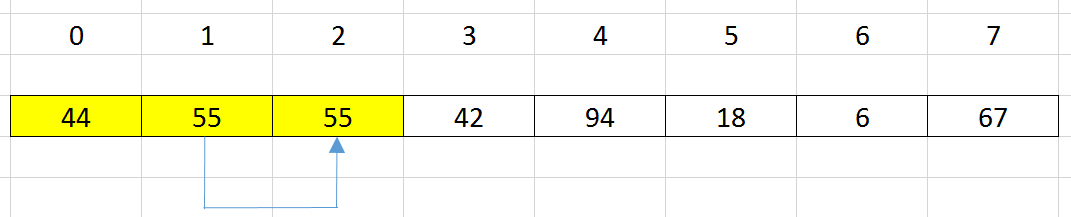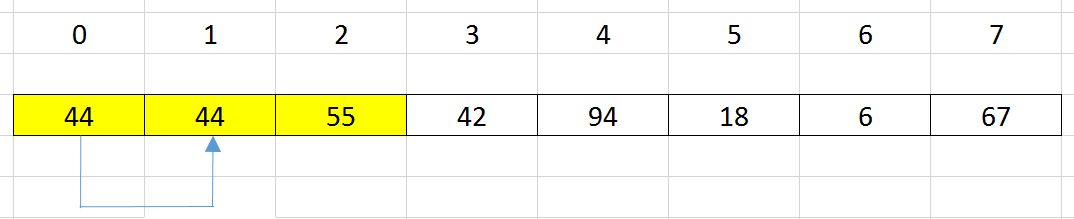• update the element’s value at index i = pos by the element’s value at index i = 2.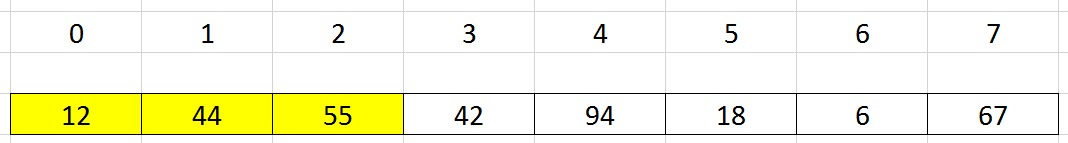4. Insert an element at index `i` = 3 into a sorted subarray has `length = 3`, from the `position = 0`.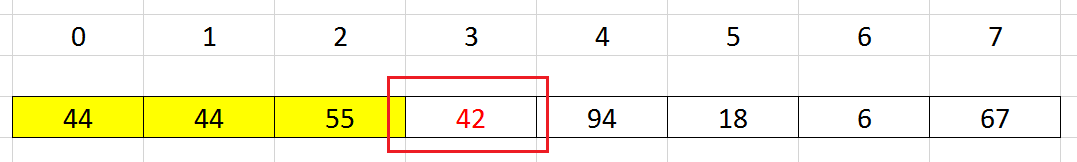• Use binary search to find position –> `pos = 1`.

• shift all elements that are from `pos` to `i - 1` to the right side by one position.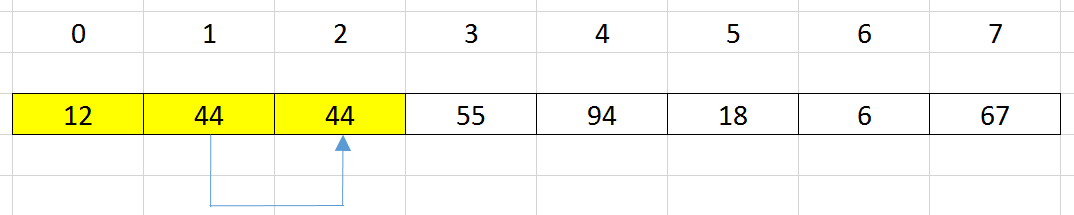• update the element’s value at index i = pos by the element’s value at index i = 3 that we’ve saved it.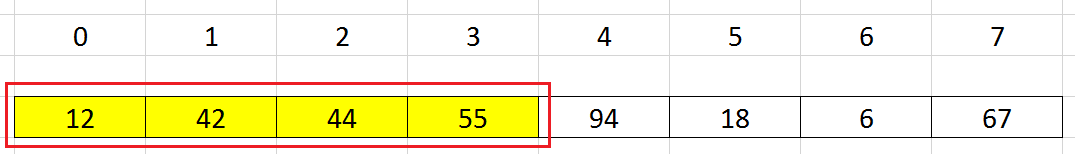5. Continue with the other elements, we can have a sorted array.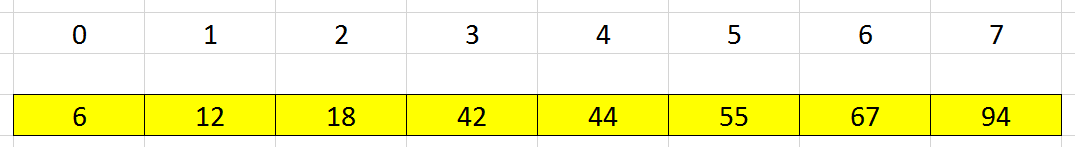The complexity of Binary Insertion sort:

• Time complexity: O(n^2)
• Space complexity: O(1)

Use Binary Insertion Sort will reduce the number of comparisons in Insertion Sort, but the number of exchange elements does not still change.

## Source code

``````public static void binaryInsertionSort(int[] nums) {
int length = nums.length;

for (int i = 1; i < length; ++i) {
int key = nums[i];
int insertedPosition = findPosition(nums, 0, i - 1, key);

for (int j = i - 1; j >= insertedPosition; --j) {
nums[j + 1] = nums[j];
}

nums[insertedPosition] = key;
}
}

public static int findPosition(int[] nums, int start, int end, int key) {
while (start <= end) {
int mid = start + (end - start) / 2;

if (key < nums[mid]) {
end = mid - 1;
} else {
start = mid + 1;
}
}

return start;
}
``````

## When to use

• When our array has the small size.

## Wrapping up

• Understanding how apply binary search with insertion sort algorithm. Note that our array is considered as a sorted array, so we can use binary search here.